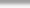## The Unix / Linux dd command: Generate, Create, Convert, Wipe and more

dd is a great unix and linux utility with many purposes and uses.  It is simply low level I/O that can ge used to generate files, convert files, wipe disks, recover disks and then some.  We've used dd quite extensively in our other posts to get work done and to recover data or disk metadata.  In this case we're looking to generate a large file in small chunks and wish to dig deeper into the inner workings of this command.  In this post we wish to demonstrate a couple of key features only.  We'll touch on a few of it's options.  We'll start with a simple file of 16 zeros:

# cat zero.txt
0000000000000000
#

Now we wish to generate a larger file in smaller chunks where we demonstrate behaviour between a few of it's options and redefine or reword the man page definition a bit:

bs=        How many bytes do you want to take out of if= to use for writing.

count=  Only works if there is a continous stream (ie if=/dev/urandom).  Otherwise anything greater then 1 is meaningless unless bs is a fraction of the if= file character count.  bs= is basically the value representing how many bytes do we wish to take out of the if= .

(1) rm skip-zero.txt; dd if=./zero.txt of=./skip-zero.txt bs=8 seek=0 count=2; strings skip-zero.txt|wc -c; wc -c skip-zero.txt  | 17, 16 skip-zero.txt
(2) rm skip-zero.txt; dd if=./zero.txt of=./skip-zero.txt bs=8 seek=0 count=1; strings skip-zero.txt|wc -c; wc -c skip-zero.txt   |  9,  8 skip-zero.txt
(3) rm skip-zero.txt; dd if=./zero.txt of=./skip-zero.txt bs=8 seek=0 count=2; strings skip-zero.txt|wc -c; wc -c skip-zero.txt  | 17, 16 skip-zero.txt

seek=  This option 'seeks' or 'moves' the write pointer X * bs.  So if bs is 16 in this case it moves 16 then writes another 16 for a total of 32 bytes.  In this case count has no effect because bs already equals in character count to if= zero.txt

(4) rm skip-zero.txt; dd if=./zero.txt of=./skip-zero.txt bs=16 seek=1 count=2; strings skip-zero.txt|wc -c; wc -c skip-zero.txt | 17, 32 skip-zero.txt

In this case we'll grow the file we created in the first example.  Note we are not removing it this time:

(5) dd if=./zero.txt of=./skip-zero.txt bs=16 seek=1 count=1; strings skip-zero.txt|wc -c; wc -c skip-zero.txt    | 33, 32 skip-zero.txt

Now we will grow it again:

(6) dd if=./zero.txt of=./skip-zero.txt bs=16 seek=2 count=1; strings skip-zero.txt|wc -c; wc -c skip-zero.txt    | 49, 48 skip-zero.txt

We can seek to a higher number but again only one stream will be written since bs= equals if= size.

(7) dd if=./zero.txt of=./skip-zero.txt bs=16 seek=4 count=1; strings skip-zero.txt|wc -c; wc -c skip-zero.txt    | 66, 80 skip-zero.txt

Let's try to sample /dev/urandom now:

(8) dd if=/dev/urandom of=./urandom.txt bs=16 seek=0 count=1; strings urandom.txt|wc -l; wc -c urandom.txt    | 0, 16 urandom.txt

Cheers,
TK